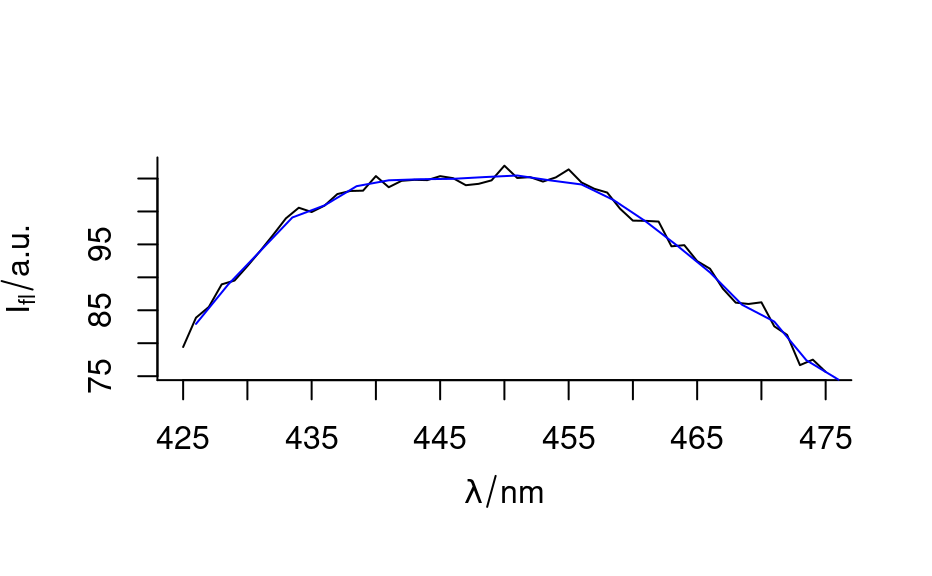In order to reduce the spectral resolution and thus gain signal to noise ratio or to reduce the dimensionality of the spectral data set, the spectral resolution can be reduced.

spc_bin(spc, by = stop("reduction factor needed"), na.rm = TRUE, ...)

Arguments

spc

The hyperSpec object.

by

Reduction factor.

na.rm

decides about the treatment of NAs:

• if FALSE or 0, the binning is done using na.rm = FALSE,

• if TRUE or 1, the binning is done using na.rm = TRUE,

• if 2, the binning is done using na.rm = FALSE, and resulting NAs are corrected with mean(..., na.rm = TRUE). See section "Details".

...

Ignored.

Value

A hyperSpec object with ceiling(nwl(spc)/by) data points per spectrum.

Details

The mean of every by data points in the spectra is calculated.

Using na.rm = TRUE always takes about twice as long as na.rm = FALSE.

If the spectra matrix does not contain too many NAs, na.rm = 2 is faster than na.rm = TRUE.

C. Beleites

Examples

spc <- spc_bin(flu, 5)
#> Warning: Last data point averages only 1 point.

plot(flu[1, , 425:475])
plot(spc[1, , 425:475], add = TRUE, col = "blue")nwl(flu)
#>  181
nwl(spc)
#>  37